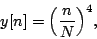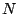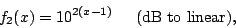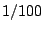Next: Continuous and discontinuous control Up: Automation and voice management Previous: Envelope Generators   Contents   Index

Linear and Curved Amplitude Shapes

Suppose you wish to fade a signal in over a period of ten seconds--that is, you wish to multiply it by an amplitude-controlling signalwhich rises from 0 to 1 in value oversamples, whereis the sample rate. The most obvious choice would be a linear ramp:. But this will not turn out to yield a smooth increase in perceived loudness. Over the first secondrises fromdB to -20 dB, over the next four by another 14 dB, and over the remaining five, only by the remaining 6 dB. Over most of the ten second period the rise in amplitude will be barely perceptible.

Another possibility would be to rampexponentially, so that it rises at a constant rate in dB. You would have to fix the initial amplitude to be inaudible, say 0 dB (if we fix unity at 100 dB). Now we have the opposite problem: for the first five seconds the amplitude control will rise from 0 dB (inaudible) to 50 dB (pianissimo); this part of the fade-in should only have taken up the first second or so.

A more natural progression would perhaps have been to regard the fade-in as a timed succession of dynamics, 0-ppp-pp-p-mp-mf-f-ff-fff, with each step taking roughly one second.

A fade-in ideally should obey some scale in between logarithmic and linear. A somewhat arbitrary choice, but useful in practice, is the quartic curve:whereis the number of samples to fade in over (in the example above, it's). So, after the first second of the ten we would have risen to -80 dB, after five seconds to -24 dB, and after nine, about -4 dB.

Figure 4.3 shows three amplitude transfer functions:The second function converts from dB to linear, arranged so that the input range, from 0 to 1, corresponds to 40 dB. (This input range of 40 dB corresponds to a reasonable dynamic range, allowing 5 dB for each of 8 steps in dynamic.) The quartic curve imitates the exponential (dB) curve fairly well for higher amplitudes, but drops off more rapidly for small amplitudes, reaching true zero at right (whereas the exponential curve only goes down to).We can think of the three curves as showing transfer functions, from an abstract control (ranging from 0 to 1) to a linear amplitude. After we choose a suitable transfer function, we can compute a corresponding amplitude control signal; if we wish to ramp oversamples from silence to unity gain, the control signal would be:A block diagram for this is shown in Figure 4.4. Here we are introducing a new type of block to represent the application of a transfer function. For now we won't worry about its implementation; depending on the function desired, this might be best done arithmetically or using table lookup.Next: Continuous and discontinuous control Up: Automation and voice management Previous: Envelope Generators   Contents   Index
Miller Puckette 2006-12-30